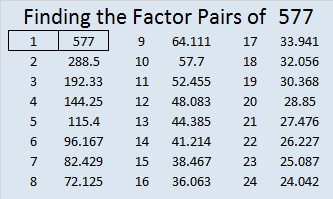# 577 and Level 6

24² + 1 = 577 means 577 is the hypotenuse of a primitive Pythagorean triple which happens to be 48-575-577.Print the puzzles or type the solution on this excel file: 10 Factors 2015-08-03

—————————————————————————————————

• 577 is a prime number.
• Prime factorization: 577 is prime.
• The exponent of prime number 577 is 1. Adding 1 to that exponent we get (1 + 1) = 2. Therefore 577 has exactly 2 factors.
• Factors of 577: 1, 577
• Factor pairs: 577 = 1 x 577
• 577 has no square factors that allow its square root to be simplified. √577 ≈ 24.020824How do we know that 577 is a prime number? If 577 were not a prime number, then it would be divisible by at least one prime number less than or equal to √577 ≈ 24.02. Since 577 cannot be divided evenly by 2, 3, 5, 7, 11, 13, 17, 19, or 23, we know that 577 is a prime number.

—————————————————————————————————This site uses Akismet to reduce spam. Learn how your comment data is processed.